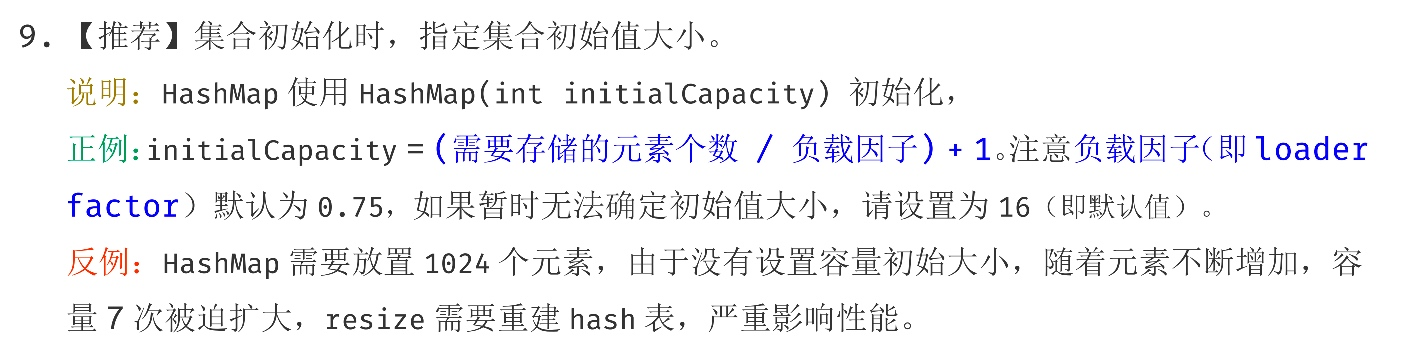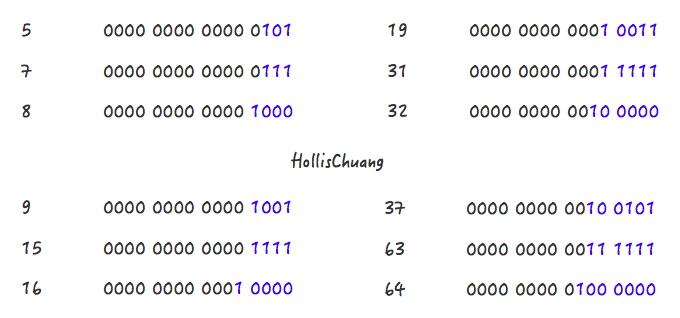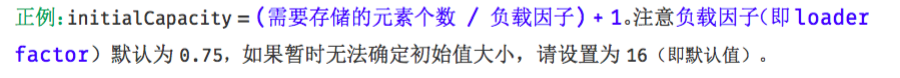# 为什么阿里巴巴建议集合初始化时，指定集合容量大小

GitHub 19k Star 的Java工程师成神之路，不来了解一下吗！### 为什么要设置HashMap的初始化容量

``````public static void main(String[] args) {
int aHundredMillion = 10000000;

Map<Integer, Integer> map = new HashMap<>();

long s1 = System.currentTimeMillis();
for (int i = 0; i < aHundredMillion; i++) {
map.put(i, i);
}
long s2 = System.currentTimeMillis();

System.out.println("未初始化容量，耗时 ： " + (s2 - s1));

Map<Integer, Integer> map1 = new HashMap<>(aHundredMillion / 2);

long s5 = System.currentTimeMillis();
for (int i = 0; i < aHundredMillion; i++) {
map1.put(i, i);
}
long s6 = System.currentTimeMillis();

System.out.println("初始化容量5000000，耗时 ： " + (s6 - s5));

Map<Integer, Integer> map2 = new HashMap<>(aHundredMillion);

long s3 = System.currentTimeMillis();
for (int i = 0; i < aHundredMillion; i++) {
map2.put(i, i);
}
long s4 = System.currentTimeMillis();

System.out.println("初始化容量为10000000，耗时 ： " + (s4 - s3));
}
``````

``````未初始化容量，耗时 ： 14419

``````

### HashMap中容量的初始化

``````Map<String, String> map = new HashMap<String, String>(1);
map.put("hahaha", "hollischuang");

Class<?> mapType = map.getClass();
Method capacity = mapType.getDeclaredMethod("capacity");
capacity.setAccessible(true);
System.out.println("capacity : " + capacity.invoke(map));
``````

``````    int n = cap - 1;
n |= n >>> 1;
n |= n >>> 2;
n |= n >>> 4;
n |= n >>> 8;
n |= n >>> 16;
return (n < 0) ? 1 : (n >= MAXIMUM_CAPACITY) ? MAXIMUM_CAPACITY : n + 1;
``````Step 1，5->7

Step 2，7->8

Step 1，9->15

Step 2，15->16

Step 1，19->31

Step 2，31->32

``````n |= n >>> 1;
n |= n >>> 2;
n |= n >>> 4;
n |= n >>> 8;
n |= n >>> 16;
``````

``````return (n < 0) ? 1 : (n >= MAXIMUM_CAPACITY) ? MAXIMUM_CAPACITY : n + 1;
``````

Step 2 比较简单，就是做一下极限值的判断，然后把Step 1得到的数值+1。

Step 1 怎么理解呢？其实是对一个二进制数依次向右移位，然后与原值取或。其目的对于一个数字的二进制，从第一个不为0的位开始，把后面的所有位都设置成1。

``````1100 1100 1100 >>>1 = 0110 0110 0110
1100 1100 1100 | 0110 0110 0110 = 1110 1110 1110
1110 1110 1110 >>>2 = 0011 1011 1011
1110 1110 1110 | 0011 1011 1011 = 1111 1111 1111
1111 1111 1111 >>>4 = 1111 1111 1111
1111 1111 1111 | 1111 1111 1111 = 1111 1111 1111
``````

``````Step 1:
0100 >>>1 = 0010
0100 | 0010 = 0110
0110 >>>1 = 0011
0110 | 0011 = 0111
Step 2:
0111 + 0001 = 1000
``````

``````int n = cap - 1;
``````

``````    int n = cap - 1;
n |= n >>> 1;
n |= n >>> 2;
n |= n >>> 4;
n |= n >>> 8;
n |= n >>> 16;
return (n < 0) ? 1 : (n >= MAXIMUM_CAPACITY) ? MAXIMUM_CAPACITY : n + 1;
``````

### HashMap中初始容量的合理值``````public static <K, V> HashMap<K, V> newHashMapWithExpectedSize(int expectedSize) {
return new HashMap<K, V>(capacity(expectedSize));
}

/**
* Returns a capacity that is sufficient to keep the map from being resized as long as it grows no
* larger than expectedSize and the load factor is ≥ its default (0.75).
*/
static int capacity(int expectedSize) {
if (expectedSize < 3) {
checkNonnegative(expectedSize, "expectedSize");
return expectedSize + 1;
}
if (expectedSize < Ints.MAX_POWER_OF_TWO) {
// This is the calculation used in JDK8 to resize when a putAll
// happens; it seems to be the most conservative calculation we
// can make.  0.75 is the default load factor.
return (int) ((float) expectedSize / 0.75F + 1.0F);
}
return Integer.MAX_VALUE; // any large value
}
``````

`return (int) ((float) expectedSize / 0.75F + 1.0F);`上面有一行注释，说明了这个公式也不是guava原创，参考的是JDK8中putAll方法中的实现的。感兴趣的读者可以去看下putAll方法的实现，也是以上的这个公式。

### 总结

``````Map<String, String> map = Maps.newHashMapWithExpectedSize(10);
``````

(全文完)### 评论 1

1. #1

您好，请问这段代码执行过程中，如果中途发生GC对于结果会有大的影响吗？

FAB\$3年前 (2019-04-15)回复

## HollisChuang's Blog

• 微信咨询
• 去评论
• 回顶
###### 回顶部